How To Make PCA Plot with R

• Last Updated : 23 Sep, 2021

Principal component analysis(PCA) in R programming is the analysis of the linear components of all existing attributes. Principal components are linear combinations (orthogonal transformation) of the original predictor in the dataset. It is a useful technique for EDA(Exploratory data analysis) and allowing you to better visualize the variations present in a dataset with many variables.

It works best with numerical data values. In this process the principal components of data are calculated and are used for performing a change of basis on the data, sometimes using only the first few principal components while ignoring the rest. One can take PCA as a kind of linear transformation of the data on the basis of certain data spaces. This transformation fits the data into a coordinate system where the most significant variance is found on the first coordinate, and each subsequent coordinate is orthogonal to the last and has a lesser variance than the previous.

PCA Plot in R

We are gonna work on the ‘Iris’ dataset, which is built into R. It is a multivariate dataset that consists of data on 50 samples from each of three species of Iris (Iris setosa, Iris virginica, and Iris versicolor).

R

 # structure of the iris# datasetstr(iris)  # print the iris datasethead(iris)

Output: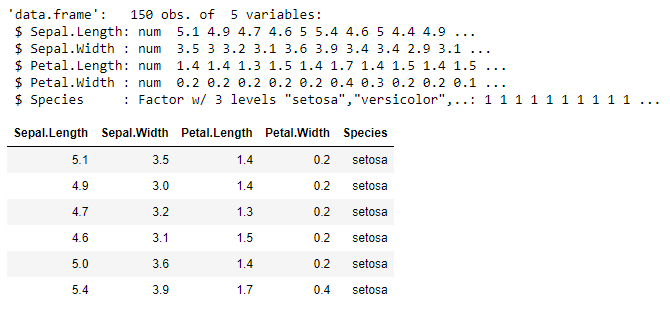As mentioned PCA works best with numerical data we will neglect the categorical variable Species. We are now left with a matrix of 4 columns and 150 rows which we will pass through prcomp( ) function for the principal component analysis. This function returns the results as an object of class ‘prcomp’. We will assign the output to a variable named iris.pca.

R

 iris.pca <- prcomp(iris[,c(1:4)],                   center = TRUE,                   scale. = TRUE)  # summary of the # prcomp objectsummary(iris.pca)

Output: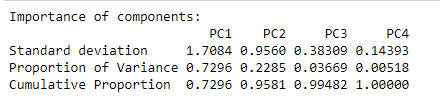Here we get four principal components named PC1-4. Each of these explains a percentage of the total variation in the dataset. For example, PC1 explains nearly 72% of the total variance i.e. around three-fourth of the information of the dataset can be encapsulated by just that one Principal Component. PC2 explains 22% and so on.

Let us take a glance at the structure of the PCA object so formed.

R

 # structure of the pca objectstr(iris.pca)

Output: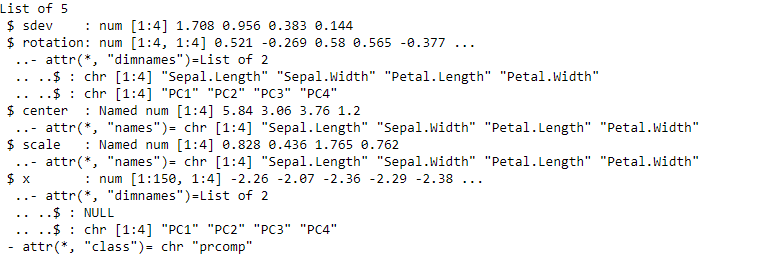Plotting PCA

While talking about plotting a PCA we generally refer to a scatterplot of the first two principal components PC1 and PC2. These plots reveal the features of data such as non-linearity and departure from normality. PC1 and PC2 are evaluated for each sample vector and plotted.

The autoplot( ) function of the ‘ggfortify package’ gives ease in plotting PCA’s in R.

R

 # loading librarylibrary(ggfortify)iris.pca.plot <- autoplot(iris.pca,                          data = iris,                          colour = 'Species')  iris.pca.plot

Output: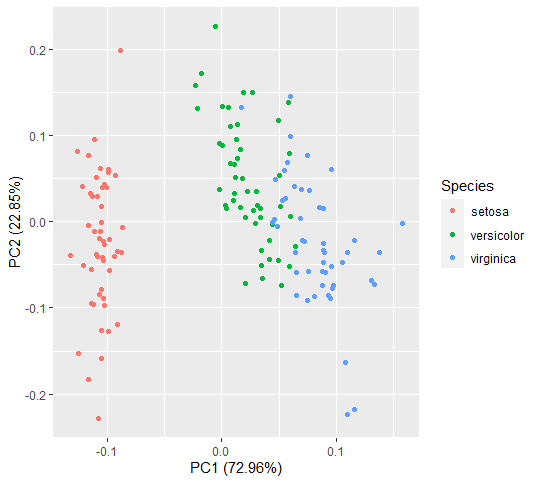For a better understanding of the linear transformation of features, biplot( ) function also be used to plot  PCA.

R

 biplot.iris.pca <- biplot(iris.pca)biplot.iris.pca

Output: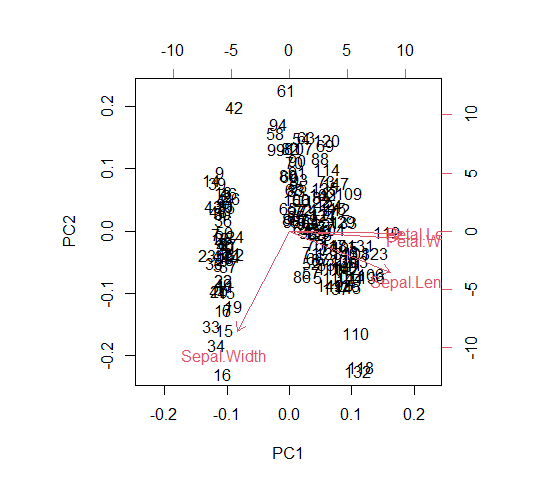The X-axis of the biplot represents the first principal component where the petal length and petal width are combined and transformed into PC1 with some parts of sepal length and sepal width. Whereas the vertical part of the sepal length and sepal width forms the second principal component.

For determining the ideal features which can be justified after performing PCA, the plot( ) function can be used to plot the precomp object.

R

 plot.iris.pca <- plot(iris.pca, type="l")plot.iris.pca

Output: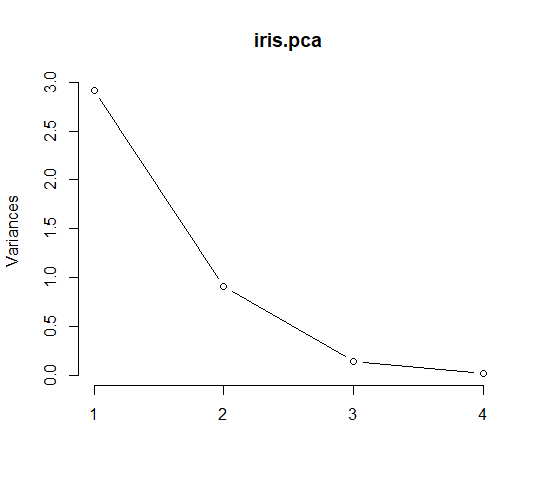In a screeplot the ‘arm-bend’ represents a decrease in cumulative contribution. The above plot shows the bend at the second principal component.

My Personal Notes arrow_drop_up